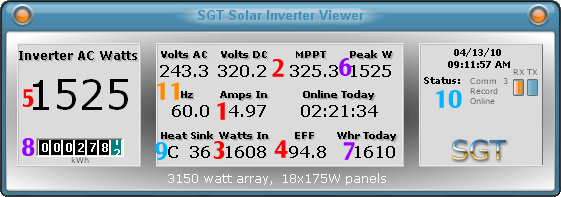# What do the “Current status” numbers mean?1. First is the amps being produced by the array. Amps is a way of measuring electricity. This number basically corresponds to the amount of sunlight hitting the array. The more sun, the more electricity.
2. Second is the volts on the line between the inverter and the array. Volts are a way of measuring the force or “pressure” of the electricity on the line. There are two numbers that are relevant here. One is the current voltage. The second is the MPPT number, or Maximum Power Point Tracker, which is basically the current target for the optimal voltage that the inverter thinks should be on the line. The optimal voltage changes depending on the amount of sunlight hitting the array, this has to do with the physics of how the solar panels produce electricity. So the inverter constantly recalculates the MPPT and changes the voltage.
3. Third is the watts being received by the inverter. Watts are an abstract way of measuring the amount of usable electricity you have. It’s how the electric company measures the amount of electricity you use. Watts is really nothing more than amps times volts. At any given moment, if you multiply the number of amps on the line by the volts on the line, it will be roughly equal to the watts being received by the inverter.
4. Fourth is the efficiency of the inverter, as a percentage. The main role of the inverter is to convert direct current (DC) electricity from the solar array into alternating current (AC) electricity that can be used in our house and on the public power grid. The efficiency percentage shows how well the inverter is doing the conversion at the moment. Efficiency is highest when there is constant, bright, direct sunlight.
5. Fifth is the watts being output by the inverter right now. If you multiply the incoming watts by the efficiency percentage, you will get the number of watts being output.

6. Sixth is the peak watts output today. This number is the highest number of watts output at any one moment today. The watt output often peaks in the middle of the day and/or at a moment when the sun moves behind the edge of a cloud, which sometimes acts like a lens.
7. Seventh is the cumulative output today, expressed in watt hours. If the system outputs 1,000 watts for two hours, that equals 2,000 watt hours. The microFIT program pays us 80.2¢ per kilowatt hour (thousand watt hours).
8. Eight is the lifetime total output, expressed in kilowatt hours. This is the total output of our inverter since it was manufactured. When we got it, there were a few kilowatts on it already due to factory testing, just like a new car’s odometer never starts at zero.

9. Ninth is the current temperature of the inverter’s heat sink. There is a large set of metal fins on the front of the inverter to dissipate heat that builds up during the DC-to-AC conversion process.
10. Tenth is the current status display. This is where the current state of the inverter is shown, usually just online or offline. Sometimes you will see special messages in this area, such as when the maximum power input is reached, or the connection countdown at sunrise (the inverter takes five minutes to come online once it detects electrical current coming from the array).

11. Eleventh is the status of the AC power coming in from the grid. The volts and hertz are shown. Hertz is the frequency of the power on the grid. These numbers remain pretty constant, especially the hertz.